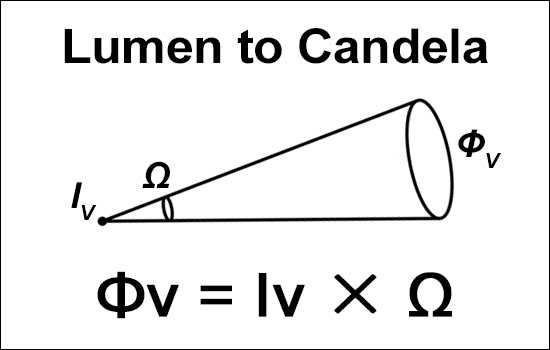# Lumen Candela Conversion Calculator

Output values are rounded to the second decimal place.

Equations

Φv = Iv * Ω

Ω = 2π(1 - cos(θπ / 360))

Φv = Luminous Flux(lm), Iv = Luminous Intensity(cd), Ω = Solid Angle of Beam in Steradians(sd), θ = Beam Angle in Degrees(°)### Input

Φv: lm

Iv:

Beam Angle:

Leave either Φv or Iv blank.